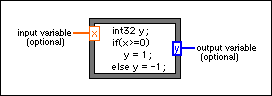# Formula Node

## LabVIEW 2018 Help

Edition Date: March 2018
Part Number: 371361R-01
View Product InfoLabVIEW 2016 HelpLabVIEW 2017 HelpLabVIEW 2018 HelpLabVIEW 2019 HelpLabVIEW 2020 Help

Owning Palette: Structures

Requires: Base Development System

Evaluates mathematical formulas and expressions similar to C on the block diagram. The following built-in functions are allowed in formulas: abs, acos, acosh, asin, asinh, atan, atan2, atanh, ceil, cos, cosh, cot, csc, exp, expm1, floor, getexp, getman, int, intrz, ln, lnp1, log, log2, max, min, mod, pow, rand, rem, sec, sign, sin, sinc, sinh, sizeOfDim, sqrt, tan, tanh. There are some differences between the parser in the Mathematics VIs and the Formula Node.Refer to Creating Formula Nodes and Formula Node Syntax for more information about the Formula Node and the Formula Node syntax. Also, keep in mind the allowed operators in the Formula Node.

Formula Nodes have the following restrictions:

• Formula Nodes accept only the period (.) as a decimal separator. The nodes do not recognize localized decimal separators.
• Formula Nodes accept only pi as a constant. The constant is case-sensitive.